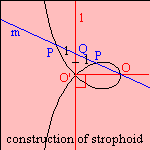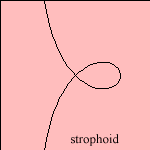# (right) strophoid

## cubicThe strophoid 1) is an extension of the cissoid 2), and in Cartesian coordinates it is written as:To construct the curve: given a line l, and a point O (called the pole), that is perpendicular projected on l in O'. Make now a line bundle m through O. The strophoid is now the collection of the points P on m for which O'Q = PQ. Q is the intersection of l and m.
This construction is closely related to the one of the cissoid, and it can also be seen as a specimen of the general cissoid.Roberval found the curve as the result of planes cutting a cone: when the plane rotates (about the tangent at its vertex) the collection of foci of the obtained conics gives the strophoid.

The strophoid has the following qualities:

• the polar inverse of the strophoid is the curve itself (with the pole as center of inversion). On the other hand, when the node is taken as center of inversion, its inverse is the hyperbola.
• the curve is a pedal of the parabola.
• the strophoid is formed by the points of contact of parallel tangents to the cochleoid.
• the curve is a nodal curve
In the middle of the 17th century mathematicians like Torricelli (1645) and Barrow (1670) studied the curve. The name strophoid was proposed by Montucci in 1846.

In the 19th century J.Booth wrote about the curve in the Proceedings of the Royal Society of Londen (vol.9, 1857-1859). He called the curve the logocyclic curve.

The curve is also named the foliate.
The strophoid is called the right strophoid to distinguish from the more general oblique strophoid.
A strophoid plane is used as a model of beta-barrels in proteins.

notes

1) Strophč (Gr.) = turn, swing
In Dutch: striklijn.

2) As the strophoid's equation can be rewritten as: r = cosφ - sinφ tanφ,
which is equivalent with the term cosφ added to the cissoid's equation.